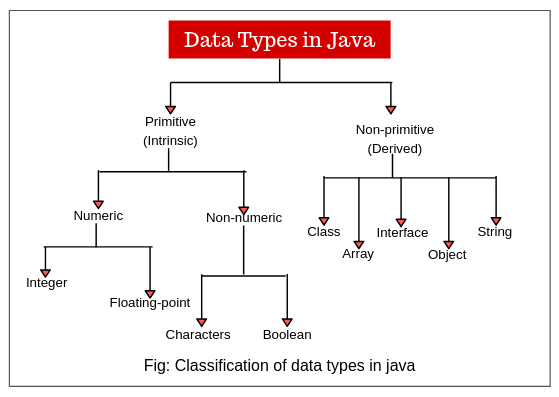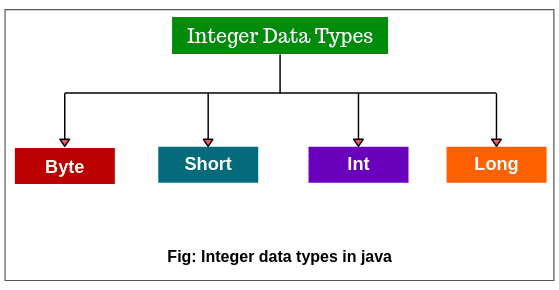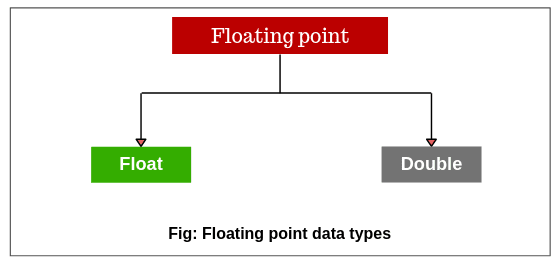# Data types in Java | Primitive Data type, Example

We know that we need a variable to store data. Internally, a variable represents a memory location where data is stored.

When we use a variable in java program, we have to declare first it as:

`int x;`

Here, “x” is a variable that can store int (integer) type data. It means that int represents the nature of data that can be stored into x. Thus, int is called data type in java.

A data type in java is a term that specifies memory size and type of values that can be stored into the memory location. In other words, data types define different values that a variable can take.

Let’s take some examples to understand data types.

1. Variable x can store an integer number like 100 as: x = 100; // Here, = represents that value 100 is stored into x.

2. String name = “Deep”; // Here, String is a data type and name is a variable that can take only string values.

3. int num = 10; // Here, int is a data type and num is variable which can only take integer values like 10, 20, 30, 40, and so on.

The semicolon is used to end a particular statement in java like a pull stop in the English language so that java could know that statement has completed.

## Types of Java Data Types

Java language provides several data types, as shown in the below figure. It divided all data types into two categorized that are as:

1. Primitive data types (also called intrinsic or built-in types)
2. Non-primitive data types (also called derived or reference data type)Numeric data types are also called arithmetic data types in java.

## Primitive Data types in Java

Primitive data types in Java are those data types whose variables can store only one value at a time. We cannot store multiple values of the same type. These data types are pre-defined in Java. They are named by a Keyword.

For example:

```int x; // valid
x = 10; // valid because "x" store only one value at a time because it is the primitive type variable.
x = 10, 20 ,30 40; // invalid.```

Primitive data types are not user-defined data-types. i.e. Programmers cannot develop primitive data types.

## Types of Primitive data types in Java

Java defines eight primitive data types: boolean, char, byte, short, int, long, char, float, and double. We can further categorize these into four groups. They are as:

• Conditional category data type: boolean
• Character category data type: char
• Integer category data types: byte, short, int, and long.
• Float category data types: float and double

All the data types are the keywords that are predefined in Java. They are in small letters.

Now it is important to understand the memory limitations which decide which data type we should use for a particular number.

For example, when we define the age of a person, the age of any person will not cross 120.

In this case, using a short data type is enough instead of using long which will take a big memory. Therefore, understand the important terms for every data type.

## Size of Data Types in Java

Each data type has some memory size defined in Java. Whenever we declare a variable with a data type, the JVM automatically defines the memory size in the RAM.

For example, if we declare int a; then JVM defines 4 bytes as the size of the memory.

### Default value:

Every primitive data type has default values defined in java. When the programmer does not declare any value to the variables, the JVM will assign default values during the object creation.

For example:

• The default value of int is 0 (zero).
• The default value of the byte is 0.

Program code 1:

```package javaProgram;
public class Test {
// Declare a variable of type int.
int x;
public static void main(String[] args)
{
// Create an object of class.
Test t = new Test();
System.out.println("Default value of x: " +t.x);
}
}
```
```Output:
Default value of x: 0
```

## Range of Data types in Java

The range of value represents the min or max value a data type can hold. When we assign the value of a variable which is not fit in the range of data type, JVM will throw an error or exception.

For example, the range of int data type is between the minimum value -2,147,483,648(2^31) and the maximum value +2,147,483,648(2^31-1).

All data types represent both positive and negative numbers. In the programming language, zero is considered a positive number.

## Integer data types in Java

Integer data types in Java represent integer numeric values or numbers with no fractional parts or decimal points. For example, 225, -56524, 0, 1045, etc. come under this category.

Integer data types are again subdivided into four types: byte, short, int, and long, as shown in the below figure.Note: All java values are signed types (positive or negative). Java does not support unsigned types. Many other computer languages, including C/C++ support both unsigned and signed integers.

### Byte Data type

1. A byte is the smallest integer data type that has the least memory size allocated. It is mostly used to save memory.

2. Byte data type is an 8 bits signed two’s complement integer.

3. The memory size of byte type is a 8-bit (i.e. 1 byte). One byte is equal to 8 bits.

4. A byte keyword represents from 0 to 127 on the positive side and from -1 to -128 on the negative side. It represents any value between -128 to 127. So, it can represent the total 256(2^8) numbers.

5. Default value of byte data type is 0.

6. Variables of type byte are especially used to flow a stream of data from network or file.

For example, we can declare a variable of type byte by using byte keyword as:

```byte b, c; // Variables b and c of type byte.
```

Let’s take an example program based on the byte data type.

Program code 2:

```package datatypePrograms;
public class ByteExample
{
public static void main(String[] args)
{
byte num = 100; // byte is 8 bit value.
System.out.println(num);
}
}```
```Output:
100```

In the preceding example program, we have declared byte data type for variable num with a value 100 which is stored into num. A byte represents any value between -128 to 127.

But when you will assign 150 values at the place of 100 to variable num, you would get a compilation problem: Type mismatch error: cannot convert from int to byte because the value is out of the range of byte type. The range of byte is -128 to +127.

### Short Data type

1. Short data type has greater memory size than byte and less than int.

2. A short data type is a 16-bit signed two’s complement integer.

3. The default memory size is allocated 16 bits, i.e. 2 bytes.

4. It represents 0 to 32767 on the positive side and on the negative side, from -1 to -32768. It represents any value between -32768 to 32767. So, it can represent a total of 65536(2^16) numbers.

5. The default value of short data type is 0.

Let’s create a program based on the short data type in Java.

Program code 3:

```package datatypePrograms;
class ShortExample
{
public static void main(String[] args)
{
short num = 200; // Here, we have stored a value 200 into a variable num of short data type.
System.out.println(num);
}
}```
```Output:
200```

We cannot use byte data type in the above example because a byte cannot hold 200 value but short can hold the value 200 because of its wider range.

### Int Data type

1. We mostly use int data type for integer values in Java programming.

2. Int data type is a 32-bit signed two’s complement integer.

3. It has a wider range from -2,147,483,648 to 2,147,483,647.

4. The memory size is 32 bits, i.e. 4 bytes.

5. The default value of int data type is 0.

Let’s create a program where we will store two values into variables a and b with data type int. Then, we will add both values and display them.

Program code 4:

```package datatypeProgram;
class IntegerExample {
public static void main(String[] args)
{
int a = 200; // Here, we have stored 200 into a which is declared as int type.
int b = 300; // 300 is stored into b which is declared as int type.
System.out.println(a+b);
}
}```
```Output:
500```

### Long Data type

1. We mostly use this data type for a huge number where int type is not large enough to store the desired value.

2. A long data type is a 64-bit signed two’s complement integer.

3. Default memory size allocated to this data type is 64 bits, i.e. 8 bytes.

4. The default value of long data type is 0.

5. It has a wide range from -9,223,372,036,854,775,808 (-2^63) to 9,223,372,036,854,775,807 (-2^63-1). Long data type is useful when big whole numbers are needed.

For example:

long num = -2334456L;

Here, we have stored -2334456 into a variable num of type long. L represents JVM will consider it as a long value and will allot 8 bytes to it.

Let’s create a program where we will calculate the distance traveled by light in 1000 days using long data type. Look at the following source code.
Program code 5:

```package datatypePrograms;
public class LongExample {
public static void main(String[] args)
{
int lightSpeed;
long days;
long seconds;
long distance;
// Speed of light in miles per sec.
lightSpeed = 186282;
days = 1000;
// Number of days.
seconds = days*24*60*60; // Convert into seconds.
distance = lightSpeed * seconds;
System.out.println("In 1000 days, distance traveled by light: " +distance + " miles");
}
}```
```Output:
In 1000 days, distance traveled by light: 16094764800000 miles```

It is clear from the above example program that the distance value could not have been held in an int variable.

### Table 1: Size and Range of Integer Data types

TypeSizeMinimum valueMaximum value
byteOne byte-128127
shortTwo bytes-32, 76832, 767
intFour bytes-2, 147, 483, 6482, 147, 483, 647
longEight bytes-9, 223, 372, 036, 854, 775, 8089, 223, 372, 036, 854, 775, 807

Whenever possible you should use smaller data types because wider data types take more time for manipulation.

For example, if you want to use int variable to store 50 value, it is advisable that you should use a byte variable instead of using int variable.

This is because it will improve the speed of execution of the program and take less memory size.

## Java Floating-Point Data Types

Floating-point data types are useful for holding numbers containing decimal points or fractional parts. For example, 3.14, -2.567, 0.00034, etc. are called floating-point numbers.

There are two kinds of floating-point data types: float and double.### Float Data type

1. A float data type is used to represent the decimal number, which can hold 6 to 7 decimal digits.

2. It is used to save memory in large arrays of floating-point numbers.

3. The float data type is a single-precision 32-bit IEE 754 floating-point.

4. The default memory size allocated for this data type is 32 bits, i.e. 4 bytes.

5. The default value float data type is 0.0f.

For example:

float num = 10.6f;

Here, if we do not write “f”, then JVM will consider as double and would have allotted 8 bytes. In this case, it will give an error ” Type mismatch: cannot convert from double to float”. But if we use f, JVM will consider it as float value and allot only 4 bytes.

### Double Data type

1. A double data type is also used to represent decimal numbers up to 15 decimal digits accurately.

2. The double data type is a double-precision 64-bit IEE 754 floating-point.

3. Memory size is 64 bits, i.e. 8 bytes, and the default value is 0.0d.

For example:

double num = 1345.6;
double distance = 1.50e9; // Here, e represents x 10 to the power.

Hence, 1.50e9 means 1.50*10^9. It is called scientific notation of representing number.

Let’s make a program where we will use double variables to calculate the area of circle.

Program code 6:

```package datatypePrograms;
public class Area {
public static void main(String[] args)
{
double pi, r;
r = 5.5; // Radius of circle.
pi = 3.1416;
// Calculate the area of circle.
double area = pi * r * r;
System.out.println("Area of circle: " +area);
}
}```
```Output:
Area of circle: 95.0334```

Note: A single-precision takes lesser space in the memory than double precision. But when the value is large, single-precision becomes inaccurate.

Therefore, double precision is the best choice when we want to store a large number. For example, we can use it for mathematical functions like sin(), cos(), sqrt().

### Table 2: Size and Range of Floating-point Data types

TypeSizeMinimum valueMaximum value
floatFour bytes3.4e-0383.4e+038
doubleEight bytes1.7e-3081.7e+308

### Character Data type

1. A char data type is mainly used to store a single character like P, a, b, z, x, etc.

2. It is a single 16-bit Unicode character.

3. Memory size taken by a single char is 2 bytes.

4. It can represent a range of 0 to 65536 characters.

5. A default value for char is ‘u0000’ which represents blank space or single space. Here, ‘u’ represents that character is a Unicode.

For example:

char ch = ‘D’;

Let’s take an example program based on the character data type.

Program code 7:

```package datatypePrograms;
public class CharExample {
public static void main(String[] args)
{
char ch1, ch2;
ch1 = 88;
ch2 = 'R';
char ch3;
ch3 = 'A';

ch3++;
System.out.println(ch1);
System.out.println(ch2);
System.out.println(ch3);
}
}```
```Output:
X
R
B```

In the above program, we have assigned a value 88 (which is an ASCII value) in a variable ch1, and specifies a letter X. ch3 is assigned value A and then it is incremented. So, ch3 will now store B, the next character in the ASCII sequence.

### Boolean Data type

1. boolean data type represents one bit of information as either true or false. i.e. there is only two possibles value true or false. Internally, JVM uses one bit of storage to represent a boolean value.

2. It is generally used to test a particular conditional statement during the execution of the program.

3. Boolean data type takes zero bytes of memory.

4. Default value is false.

For example:

boolean b = false;

In the above all examples, we assigned a value of the variable, assigned value will be printed as output.

Suppose if you do not assign the value of the variable, JVM will assign the default value and the default value will be print. Let’s take an example program based on boolean data type.

Program code 8:

```package datatypePrograms;
public class DefaultExample
{
// Declaration of instance Variables.
int a;
char b;
float c;
boolean d;
// Static method or main method.
public static void main(String[] args)
{
// Create the object of the class.
DefaultExample obj = new DefaultExample();
// Call the variable and print it.
System.out.println(obj.a);
System.out.println(obj.b);
System.out.println(obj.c);
System.out.println(obj.d);
}
}```
```Output:
0
u0000 (represents blank space)
0.0
false```

## Why take boolean data types zero bytes of memory?

Boolean data type takes zero bytes of memory space because boolean data type in Java is implemented by Sun Micro System using the concept of a flip-flop.

A flip-flop is a general-purpose register that stores one bit of information (one for true and zero for false).

## Different ways to initialize values:

```1. int a = 10; // Initialization.
System.out.println(a);
Output: 10

2. int a, b, c; // Initialization.
System.out.println(a);
System.out.println(b);
System.out.println(c);
Output: o, o, o

3. int a = 20, b, c;
System.out.println(a);
System.out.println(b);
System.out.println(c);
Output: 20, 0, 0

4. int a = 10, b = 20, c;
System.out.println(a);
System.out.println(b);
System.out.println(c);
Output: 10, 20, 0

5. int a = 10, b = 20, c = 30;
Output: 10, 20, 30```

Key Points:

1. Primitive data types in Java programming is used to store a single value. It is also known as fundamental data types.

2. Non-primitive data types known as advanced data types store several values or a group of values.

3. The basic and most common data types are integer, floating-point number, character, string, and boolean.

4. Data types are used in java because java is a strongly typed language. Java compiler checks type compatibility. Illegal operations cannot be compiled.

In this tutorial, you learned data types in Java with with example programs. Hope that you will have understood types of primitive data types in Java.

In the next tutorial, we will learn Java reference data types.Next ⇒ Non-primitive data types in Java